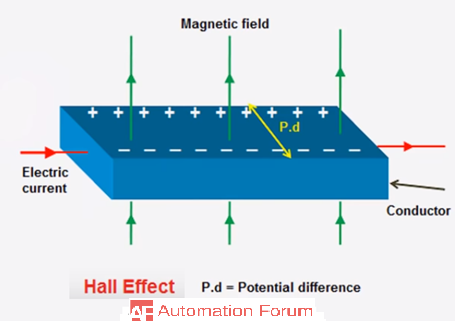# Hall effect and its applications

What is hall effect

The effect of generating measurable voltage by using a magnetic field is called a hall-effect it’s a phenomenon which occurs in a current-carrying conductor when it is placed in a magnetic field perpendicular to the current. The charge carriers in the conductor become deflected by the magnetic field and give rise to an electric field, which will be perpendicular to both the current and magnetic field. Hall-effect is very useful for many applications and devices such as magnetic field measurements, position and motion detectors. It is also useful for characterizing the electrical transport properties of metals and semiconductors. Hall-effect sensors can be used for any purpose such as rotating speed sensor, fluid flow sensor, current and pressure sensors. The hall-effect transducer can be used to measure a magnetic field and convert it into a measurable voltage.What is hall voltage

If a strip of conducting material carries a current in the presence of transverse magnetic field a potential difference will be produced between the opposite edges of the conductor and the electric field which is created by this will be perpendicular to both the current and magnetic field. The voltage or electric field produced due to the application of a magnetic field is called as hall voltage or hall field. Hall voltage is directly proportional to the current flow of the material, the magnetic field strength is inversely proportional to the mobile charges in the material and the thickness of the material, so if we need to produce large hall voltage we need to use thin material with few mobile charges per unit volume.

What is hall probe

It is a device which is used to measure the hall-voltage which subsequently gives the strength of a magnetic field. Conventional hall probes were constructed by using thin sheets of copper, nowadays it is constructed by using semiconductors like silicon and germanium to make it more sophisticated. Hall probe is inserted in the area where the magnetic field strength is to be determined and the hall probes give the value of the hall voltage across its two ends, then we can calculate the magnetic field strength.

Hall-voltage(VH) = IB/ned

I = current across the hall probe

B= magnetic field to be measured

n= charge density (no of charge carriers per unit volume)

e= 1.6×1019 C

d= thickness of the Hall probe

What is a quantum hall effect

The quantum hall effect is a quantum mechanical version of a hall effect. Which is observed in a two-dimensional system of electrons subjected to low temperatures and strong magnetic fields and these system doesn’t occur naturally but by using advanced technology and production techniques developed in semiconductor electronics. In the presence of large magnetic field strength and low temperature, the quantum hall effect can be observed, which is the quantization of the Hall resistance

Hall-effect in semiconductors

If a semiconductor which is current-carrying is placed in a magnetic field the carriers of charge in the semiconductor will experience a force and it will be perpendicular to both the current and magnetic field. In a semiconductor, the electrons will move along a closed trajectory but with strong magnetic fields and at normal temperatures this movement is fragmented by collisions. The charge carriers present in a semiconductor which is electrons and holes their concentration and mobility will change because of this effect.

Hall effect can be used to determine if a semiconductor is p-type or n-type, if the produced voltage in a semiconductor is positive then it is a p-type semiconductor and if the produced voltage is negative then it is an n-type semiconductor.

Hall measurement and resistivity measurement

To determine the mobility and the sheet density hall and resistivity measurement are needed. The main objective of the resistivity measurement is to determine the sheet resistance and there are two characteristics resistance according to van der Pauw. Hall measurement is done to obtain the sheet carrier density. Hall measurement can be done as the same method as hall voltage measurement by using a constant current and constant magnetic field which is applied perpendicular to the plane of the sample. Certain practical aspects must be considered while doing hall and resistivity measurements they are ohmic contact quality and size, sample uniformity and accurate thickness determination, thermomagnetic effects due to non-uniform temperature and photoconductive and photovoltaic effects.

What are the applications of hall-effect

• It can be used to find whether a semiconductor is p-type or n-type
• Carrier concentration can be found
• Mobility of the charge carriers can be calculated
• Measurement of conductivity
• AC power and strength of the magnetic field can be measured
1 Like